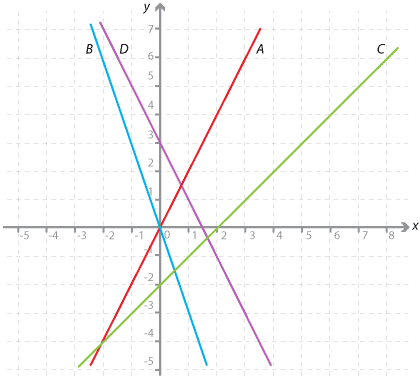#### Question 1

State the gradient and y-intercept of each line:

a b y = 2x + 6 Gradient = y-intercept = y = –x + 11 Gradient = y-intercept = y = –2x + 6 Gradient = y-intercept =

Please fill in both values before checking the answer.

#### Question 2Detailed description

Write the equation of each line shown in the diagram.

a The equation of line A is y = x + The equation of line B is y = x + The equation of line C is y = x - The equation of line D is y = x +

Please fill in both values before checking the answer.